• Posts tagged "OO"

# Blog Archives

## R语言基于R6的面向对象编程

R的极客理想系列文章，涵盖了R的思想，使用，工具，创新等的一系列要点，以我个人的学习和体验去诠释R的强大。

R语言作为统计学一门语言，一直在小众领域闪耀着光芒。直到大数据的爆发，R语言变成了一门炙手可热的数据分析的利器。随着越来越多的工程背景的人的加入，R语言的社区在迅速扩大成长。现在已不仅仅是统计领域，教育，银行，电商，互联网….都在使用R语言。

• 张丹(Conan), 程序员Java,R,PHP,Javascript
• weibo：@Conan_Z
• blog: http://blog.fens.me
• email: bsspirit@gmail.com

http://blog.fens.me/r-class-r6/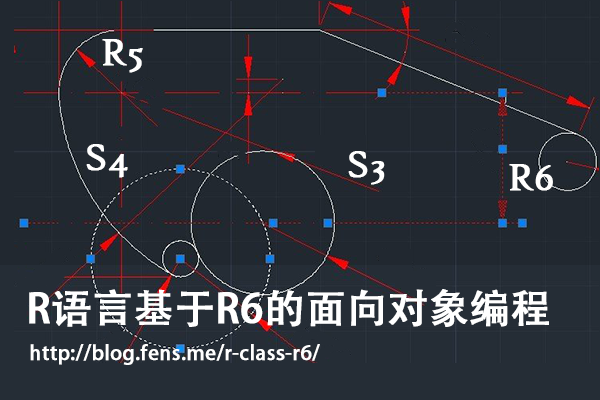R6是什么？听说过S3、S4和RC(R5)的面向对象类型 ，R6难道是一种新的类型吗？

1. 初识R6
2. 创建R6类和实例化对象
3. R6类的主动绑定
4. R6类的继承关系
5. R6类的对象的静态属性
6. R6类的可移植类型
7. R6类的动态绑定
8. R6类的打印函数
9. 实例化对象的存储
10. R6面向对象系统的案例

## 1. 初识R6

R6是一个单独的R包，与我们熟悉的原生的面向对象系统类型S3,S4RC类型不一样。在R语言的面向对象系统中，R6类型与RC类型是比较相似的，但R6并不基于S4的对象系统，因此我们在用R6类型开发R包的时候，不用依赖于methods包，而用RC类型开发R包的时候则必须设置methods包的依赖，在发布gridgame游戏包 文章中，就会出现RC依赖于methods包的使用情况。

R6类型比RC类型更符合其他编程对于面向对象的设置，支持类的公有成员和私有成员，支持函数的主动绑定，并支持跨包的继承关系。由于RC类型的面向对象系统设计并不彻底，所以才会有R6这样的包出现。下面就让我们体会一下，基于R6面向对象系统编程吧。

## 2. 创建R6类和实例化对象

• Win7 64bit
• R: 3.1.1 x86_64-w64-mingw32/x64 (64-bit)

``````
~ R                         # 启动R程序
> install.packages("R6")    # 安装R6包
> library(R6)               # 加载R6包
> library(pryr)             # 加载pryr包
``````

2.1 如何创建R6类？

R6对象系统是以类为基本类型， 有专门的类的定义函数 R6Class() 和 实例化对象的生成方法，下面我们用R6对象系统创建一个类。

``````
> R6Class
function (classname = NULL, public = list(), private = NULL,
active = NULL, inherit = NULL, lock = TRUE, class = TRUE,
portable = TRUE, parent_env = parent.frame())
``````

• classname 定义类名。
• public 定义公有成员，包括公有方法和属性。
• private 定义私有成员，包括私有方法和属性。
• active 主动绑定的函数列表。
• inherit 定义父类，继承关系。
• lock 是否上锁，如果上锁则用于类变量存储的环境空间被锁定，不能修改。
• class 是否把属性封装成对象，默认是封装，如果选择不封装，类中属性存存在一个环境空间中。
• portable 是否为可移植类型，默认是可移植型类，类中成员访问需要用调用self和private对象。
• parent_env 定义对象的父环境空间。

2.2 创建R6的类和实例化对象

``````
> Person <- R6Class("Person",    # 定义一个R6类
+  public=list(
+    hello = function(){         # 定义公有方法hello
+      print(paste("Hello"))
+    }
+  )
+)

> Person                   # 查看Person的定义
<Person> object generator
Public:
hello: function
Parent env:
Lock: TRUE
Portable: TRUE

> class(Person)             # 检查Person的类型
 "R6ClassGenerator"
``````

``````
> u1<-Person\$new()   # 实例化一个Person对象u1
> u1                 #查看u1对象
<Person>
Public:
hello: function
> class(u1)           # 检查u1的类型
 "Person" "R6"
``````

``````
> otype(Person)   # 查看Person类型
 "S3"
> otype(u1)       # 查看u1类型
 "S3"
``````

2.3 公有成员和私有成员

``````
> Person <- R6Class("Person",
+  public=list(
+    name=NA,                           # 公有属性
+    initialize = function(name){       # 构建函数方法
+      self\$name <- name
+    },
+    hello = function(){                # 公有方法
+      print(paste("Hello",self\$name))
+    }
+  )
+)

> conan <- Person\$new('Conan')          # 实例化对象
> conan\$hello()                         # 调用用hello()方法
 "Hello Conan"
``````

``````
> Person <- R6Class("Person",
+   public=list(                       # 公有成员
+     name=NA,
+     initialize = function(name,gender){
+       self\$name <- name
+       private\$gender<- gender        # 给私有属性赋值
+     },
+     hello = function(){
+       print(paste("Hello",self\$name))
+       private\$myGender()             # 调用私有方法
+     }
+   ),
+   private=list(                      # 私有成员
+     gender=NA,
+     myGender=function(){
+       print(paste(self\$name,"is",private\$gender))
+     }
+   )
+ )
> conan <- Person\$new('Conan','Male')         # 实例化对象
> conan\$hello()                               # 调用用hello()方法
 "Hello Conan"
 "Conan is Male"
``````

``````
> conan\$name            # 公有属性
 "Conan"
> conan\$gender          # 私有属性
NULL
> conan\$myGender()      # 私有方法
Error: attempt to apply non-function
``````

``````
> Person <- R6Class("Person",
+   public=list(
+     name=NA,
+     initialize = function(name,gender){
+       self\$name <- name
+       private\$gender<- gender
+     },
+     hello = function(){
+       print(paste("Hello",self\$name))
+       private\$myGender()
+     },
+     member = function(){              # 用于测试的方法
+       print(self)
+       print(private)
+       print(ls(envir=private))
+     }
+   ),
+   private=list(
+     gender=NA,
+     myGender=function(){
+       print(paste(self\$name,"is",private\$gender))
+     }
+   )
+ )
>
> conan <- Person\$new('Conan','Male')
> conan\$member()                            # 执行member()方法
<Person>                                    # print(self)的输出
Public:
hello: function
initialize: function
member: function
name: Conan

<environment: 0x0000000008cfc918>          # print(private)的输出
 "gender"   "myGender"                  # print(ls(envir=private))的输出
``````

## 3. R6类的主动绑定

``````
> Person <- R6Class("Person",
+   public = list(
+     num = 100
+   ),
+   active = list(                      # 主动绑定
+     active  = function(value) {
+       if (missing(value)) return(self\$num +10 )
+       else self\$num <- value/2
+     },
+     rand = function() rnorm(1)
+   )
+)

> conan <- Person\$new()
> conan\$num                   # 查看公有属性
 100
> conan\$active                # 调用主动绑定的active()函数，结果为 num +10= 100+10=100
 110
``````

``````
> conan\$active<-100    # 传参数
> conan\$num            # 查看公有属性num
 50
> conan\$active         # 调用主动绑定的active()函数，结果为 num+10=50+10=60
 60
> conan\$active(100)    # 如果进行方法调用，其实会提示没有这个函数的
Error: attempt to apply non-function
``````

``````
> conan\$rand           # 调用rand函数
 -0.4767338
> conan\$rand
 0.1063623
> conan\$rand<-99       # 传参出错
Error in (function ()  : unused argument (quote(99))
``````

## 4. R6类的继承关系

``````
> Person <- R6Class("Person",
+   public=list(                            # 公有成员
+     name=NA,
+     initialize = function(name,gender){
+       self\$name <- name
+       private\$gender <- gender
+     },
+     hello = function(){
+       print(paste("Hello",self\$name))
+       private\$myGender()
+     }
+   ),
+   private=list(                           # 私有成员
+     gender=NA,
+     myGender=function(){
+       print(paste(self\$name,"is",private\$gender))
+     }
+   )
+ )
``````

``````
> Worker <- R6Class("Worker",
+   inherit = Person,                # 继承，指向父类
+   public=list(
+     bye = function(){
+       print(paste("bye",self\$name))
+     }
+   )
+ )
``````

``````
> u1<-Person\$new("Conan","Male")        # 实例化父类
> u1\$hello()
 "Hello Conan"
 "Conan is Male"

> u2<-Worker\$new("Conan","Male")        # 实例化子类
> u2\$hello()
 "Hello Conan"
 "Conan is Male"
> u2\$bye()
 "bye Conan"
``````

``````
> Worker <- R6Class("Worker",
+   inherit = Person,
+   public=list(
+     bye = function(){
+       print(paste("bye",self\$name))
+     }
+   ),
+   private=list(
+     gender=NA,
+     myGender=function(){
+       print(paste("worker",self\$name,"is",private\$gender))
+     }
+   )
+ )
``````

``````
> u2<-Worker\$new("Conan","Male")
> u2\$hello()                    # 调用hello()方法
 "Hello Conan"
 "worker Conan is Male"
``````

``````
> Worker <- R6Class("Worker",
+   inherit = Person,
+   public=list(
+     bye = function(){
+       print(paste("bye",self\$name))
+     }
+   ),
+   private=list(
+     gender=NA,
+     myGender=function(){
+       super\$myGender()                                      # 调用父类的方法
+       print(paste("worker",self\$name,"is",private\$gender))
+     }
+   )
+ )

> u2<-Worker\$new("Conan","Male")
> u2\$hello()
 "Hello Conan"
 "Conan is Male"
 "worker Conan is Male"
``````

## 5. R6类的对象的静态属性

``````
> A <- R6Class("A",
+  public=list(
+    x = NULL
+  )
+ )
>
> B <- R6Class("B",
+  public = list(
+    a = A\$new()
+  )
+ )
``````

``````
> b <- B\$new()         # 实例化B对象
> b\$a\$x <- 1           # 给x变量赋值
> b\$a\$x                # 查看x变量的值
 1

> b2 <- B\$new()        # 实例化b2对象
> b2\$a\$x <- 2          # 给x变量赋值
> b2\$a\$x               # 查看x变量的值
 2

> b\$a\$x                # b实例的a对象的x值也发生改变
 2
``````

``````
> C <- R6Class("C",
+  public = list(
+    a = NULL,
+    initialize = function() {
+      a <<- A\$new()
+    }
+  )
+ )

> cc <- C\$new()
> cc\$a\$x <- 1
> cc\$a\$x
 1

> cc2 <- C\$new()
> cc2\$a\$x <- 2
> cc2\$a\$x
 2

> cc\$a\$x        # x值未发生改变
 1
``````

## 6. R6类的可移植类型

• 可移植类型支持跨R包的继承；不可移植类型，在跨R包继承的时候，兼容性不太好。
• 可移植类型必须要用self对象和private对象来访问类中的成员，如self\$x,private\$y；不可移植类型，可以直接使用变量x,y，并通过<<-实现赋值。

``````
> RC <- setRefClass("RC",                  # RC类型的定义
+   fields = list(x = 'Hello'),
+   methods = list(
+     getx = function() x,
+     setx = function(value) x <<- value
+   )
+ )
> rc <- RC\$new()
> rc\$setx(10)
> rc\$getx()
 10
``````

``````
> NR6 <- R6Class("NR6",                # R6不可移植类型
+   portable = FALSE,
+   public = list(
+     x = NA,
+     getx = function() x,
+     setx = function(value) x <<- value
+   )
+ )
> np6 <- NR6\$new()
> np6\$setx(10)
> np6\$getx()
 10
``````

``````
> PR6 <- R6Class("PR6",
+   portable = TRUE,            # R6可移植类型
+   public = list(
+    x = NA,
+    getx = function() self\$x,
+    setx = function(value) self\$x <- value
+   )
+ )
> pr6 <- PR6\$new()
> pr6\$setx(10)
> pr6\$getx()
 10
``````

## 7. R6类的动态绑定

R6包已考虑这个情况，提供了一种动态设置成员变量的方法用\$set()函数。

``````
> A <- R6Class("A",
+   public = list(
+     x = 1,
+     getx = function() x
+   )
+ )
> A\$set("public", "getx2", function() self\$x*2)     # 动态增加getx2()方法
> s <- A\$new()
> s                     # 查看实例化对象的结构
<A>
Public:
getx: function
getx2: function
x: 1
> s\$getx2()             # 调用getx2()方法
 20
``````

``````
> A\$set("public", "x", 10, overwrite = TRUE)     # 动态改变x属性
> s <- A\$new()
> s\$x                                            # 查看x属性
 10
> s\$getx()                                       # 调用getx()方法，可移植类型x变量丢失
``````

``````
> A <- R6Class("A",
+  public = list(
+    x = 1,
+    getx = function() self\$x     # 修改为self\$x
+  )
+ )
> A\$set("public", "x", 10, overwrite = TRUE)
> s <- A\$new()
> s\$x
 10
> s\$getx()                        # 调用getx()方法
 10
``````

## 8. R6类的打印函数

R6提供了用于打印的默认方法print()，每当要打印实例化对象时，都会调用这个默认的print()方法，有点类似于Java类中默认的toString()方法。

``````
> A <- R6Class("A",
+  public = list(
+    x = 1,
+    getx = function() self\$x
+  )
+ )
> a <- A\$new()
> print(a)             # 使用默认的打印方法
<A>
Public:
getx: function
x: 1
``````

``````
> A <- R6Class("A",
+    public = list(
+      x = 1,
+      getx = function() self\$x,
+      print = function(...) {
+        cat("Class <A> of public ", ls(self), " :", sep="")
+        cat(ls(self), sep=",")
+        invisible(self)
+      }
+    )
+ )
> a <- A\$new()
> print(a)
Class <A> of public getxprintx :getx,print,x
``````

## 9. 实例化对象的存储

R6是基于S3面向对象系统的构建，而S3类型又是一种比较松散的类型，会造成用户环境空间的变量泛滥的问题。R6提供了一种方式，设置R6Class()的class参数，把类中定义的属性和方法统一存储到一个S3对象中，这种方式是默认的。另一种方式为，把把类中定义的属性和方法统一存储到一个单独的环境空间中。

``````
> A <- R6Class("A",
+  class=TRUE,
+  public = list(
+    x = 1,
+    getx = function() self\$x
+  )
+ )
> a <- A\$new()
> class(a)
 "A"  "R6"
> a
<A>
Public:
getx: function
x: 1
``````

``````
> B <- R6Class("B",
+   class=FALSE,
+   public = list(
+     x = 1,
+     getx = function() self\$x
+   )
+ )
> b <- B\$new()
> class(b)
 "environment"
> b
<environment: 0x000000000d83c970>
> ls(envir=b)
 "getx" "x"
``````

``````
> A <- R6Class("A",
+   lock=TRUE,       # 锁定环境空间
+   public = list(
+     x = 1
+   )
+ )
> s<-A\$new()
> ls(s)         # 查看s环境空间的变量
 "x"
> s\$aa<-11      # 增加新变量，错误
Error in s\$aa <- 11 : cannot add bindings to a locked environment
> rm("x",envir=s)       # 删除原有变量，错误
Error in rm("x", envir = s) :
cannot remove bindings from a locked environment
``````

``````
> A <- R6Class("A",
+  lock=FALSE,         # 不锁定环境空间
+  public = list(
+    x = 1
+  )
+ )
> s<-A\$new()
> ls(s)         # 查看s环境空间的变量
 "x"
> s\$aa<-11      # 增加变量
> ls(s)
 "aa" "x"
> rm("x",envir=s)    # 删除变量
> ls(s)
 "aa"
``````

## 10. R6面向对象系统的案例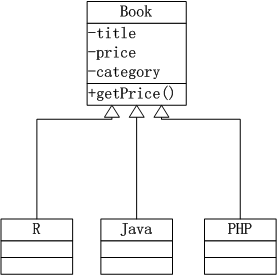``````
> Book <- R6Class("Book",            # 父类
+    private = list(
+      title=NA,
+      price=NA,
+      category=NA
+    ),
+   public = list(
+     initialize = function(title,price,category){
+       private\$title <- title
+       private\$price <- price
+       private\$category <- category
+     },
+     getPrice=function(){
+       private\$price
+     }
+   )
+ )

> R <- R6Class("R",     # 子类R图书
+    inherit = Book
+ )
> Java <- R6Class("JAVA",  # 子类JAVA图书
+   inherit = Book
+ )
> Php <- R6Class("PHP",    # 子类PHP图书
+   inherit = Book
+ )
``````

``````
> r1<-R\$new("R的极客理想-工具篇",59,"R")
> r1\$getPrice()
 59

> j1<-Java\$new("Java编程思想",108,"JAVA")
> j1\$getPrice()
 108

> p1<-Java\$new("Head First PHP & MySQL",98,"PHP")
> p1\$getPrice()
 98
``````

• 所有图书9折
• JAVA图书7折，不支持重复打折
• 为了推动R图书的销售，R语言图书7折，并支持重复打折
• PHP图书无特别优惠

``````
> Book <- R6Class("Book",
+   private = list(
+     title=NA,
+     price=NA,
+     category=NA
+   ),
+   public = list(
+     initialize = function(title,price,category){
+       private\$title <- title
+       private\$price <- price
+       private\$category <- category
+     },
+     getPrice=function(){
+       p<-private\$price*self\$discount()
+       print(paste("Price:",private\$price,", Sell out:",p,sep=""))
+     },
+     discount=function(){
+       0.9
+     }
+   )
+ )
``````

3个子类，分别对应自己的打折规则，分别进行修改。

• 给JAVA子类增加 discount()方法，用于覆盖父类的discount()方法，让JAVA图书7折，不支持重复打折，从而满足第二条规则。
• 给R子类增加 discount()方法，在子类的discount()方法中调用父类的discount()方法，让支持 R图书7折和9折的折上折，从而满足第三条规则。
• PHP子类，没有修改，完全遵循第一条规则的。
• ``````
> Java <- R6Class("JAVA",
+   inherit = Book,
+   public = list(
+     discount=function(){
+       0.7
+     }
+   )
+ )
>
> R <- R6Class("R",
+   inherit = Book,
+   public = list(
+     discount=function(){
+       super\$discount()*0.7
+     }
+   )
+ )
>
> Php <- R6Class("PHP",
+   inherit = Book
+ )
``````

分别查看3本图书的折后价格。

``````
> r1<-R\$new("R的极客理想-工具篇",59,"R")
> r1\$getPrice()
 "Price:59, Sell out:37.17"   # 59 * 0.9 *0.7= 37.17
>
> j1<-Java\$new("Java编程思想",108,"JAVA")
> j1\$getPrice()
 "Price:108, Sell out:75.6"    # 108 *0.7= 75.6
>
> p1<-Php\$new("Head First PHP & MySQL",98,"PHP")
> p1\$getPrice()
 "Price:98, Sell out:88.2"      # 98 *0.9= 88.2
``````

R图书打折最多，享受7折和9折的折上折优惠， 59 * 0.9 * 0.7= 37.17；Java图书享受7折优惠，108 *0.7= 75.6；PHP图书享受9折优惠 98 *0.9= 88.2。

通过这个实例，我们用R6的方法实现了面向对象编程中的封装、继承和多态的3个特征，证明R6是一种完全的面向对象的实现。R6类对象系统，提供了一种可兼容的面向对象实现方式，更接近于其他的编程语言上的面向对象的定义，由于R6底层基于S3来实现的，所以比RC的类更加有效果。

我们一共介绍了4种R语言的面向对象体系结构，选择自己理解的，总有一种会适合你。

转载请注明出处：
http://blog.fens.me/r-class-r6/## R语言游戏框架设计

R的极客理想系列文章，涵盖了R的思想，使用，工具，创新等的一系列要点，以我个人的学习和体验去诠释R的强大。

R语言作为统计学一门语言，一直在小众领域闪耀着光芒。直到大数据的爆发，R语言变成了一门炙手可热的数据分析的利器。随着越来越多的工程背景的人的加入，R语言的社区在迅速扩大成长。现在已不仅仅是统计领域，教育，银行，电商，互联网….都在使用R语言。

• 张丹(Conan), 程序员Java,R,PHP,Javascript
• weibo：@Conan_Z
• blog: http://blog.fens.me
• email: bsspirit@gmail.com1. 贪食蛇的面向对象改造
2. 游戏框架定义
3. 在框架中重新实现贪食蛇游戏

## 1. 贪食蛇的面向对象改造

1.1 定义Snake类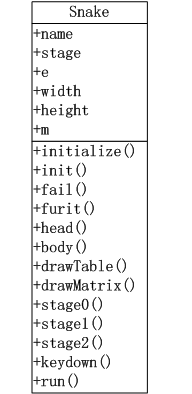• name:游戏的名字
• stage:当前的游戏场景
• e:游戏中的变量，environment类型
• m:游戏地图矩阵
• width:矩阵的宽
• height:矩阵的高

• initialize():构建函数，用于RC类的初始化
• init():给stage1场景初始化游戏变量
• fail():失败查询
• furit():判断并生成水果坐标
• body():生成蛇尾移动坐标。
• drawTable():绘制游戏背景。
• drawMatrix():绘制游戏矩阵。
• stage0():创建开机场景，可视化输出。
• stage1():创建游戏场景，stage1()函数内部，封装了游戏场景运行时的函数，并进行调用。
• stage2():创建结束场景，可视化输出
• keydown():监听键盘事件。
• run():启动函数

1.2 全局函数调用顺序图

1. 通过run()函数启动游戏，进入stage0场景，注册键盘事件。
2. 在stage0场景按任意键切换到stage1场景。
3. init()出始化stage1场景的游戏变量。
4. stage1()运行游戏
5. 当游戏失败fail()或按q键
6. 游戏进行stage2场景，显示游戏结束画面，
7. 按空格键回到stage0重新开始，按q键退出程序。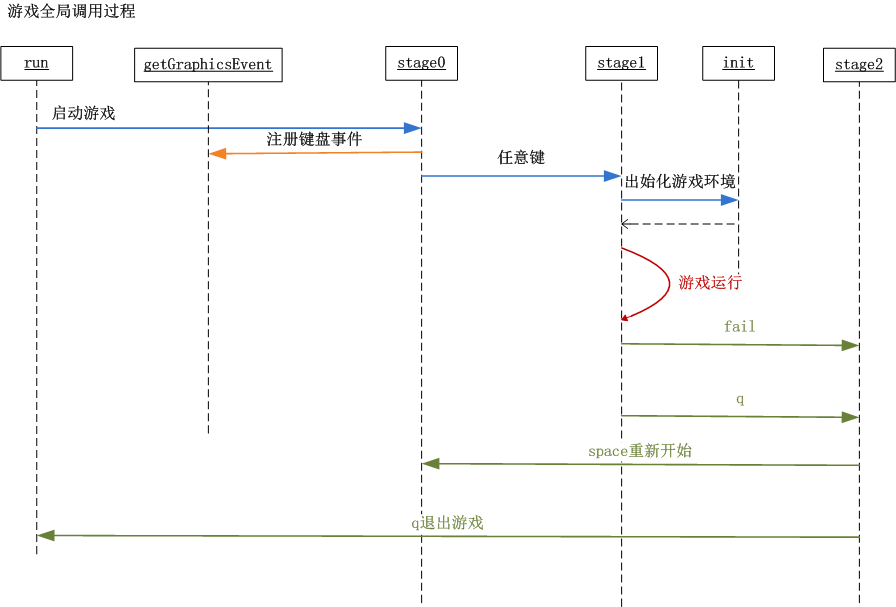1.3 stage1场景游戏环境函数调用顺序图

stage1场景游戏环境函数调用关系。

1. 游戏进入stage1场景，按上下左右(up,down,left,right)方向键操作蛇头的前进路线。
2. furit()函数检查，如果地图上水果被吃掉，生成一个新水果，记录到矩阵中。
4. fail()函数失败检查，no未失败继续，yes失败进行stage2场景。
5. body()函数，蛇身体移动，记录到矩阵中。
6. drawTable()函数，画出游戏背景画布。
7. drawMatrix()函数，画出游戏矩阵。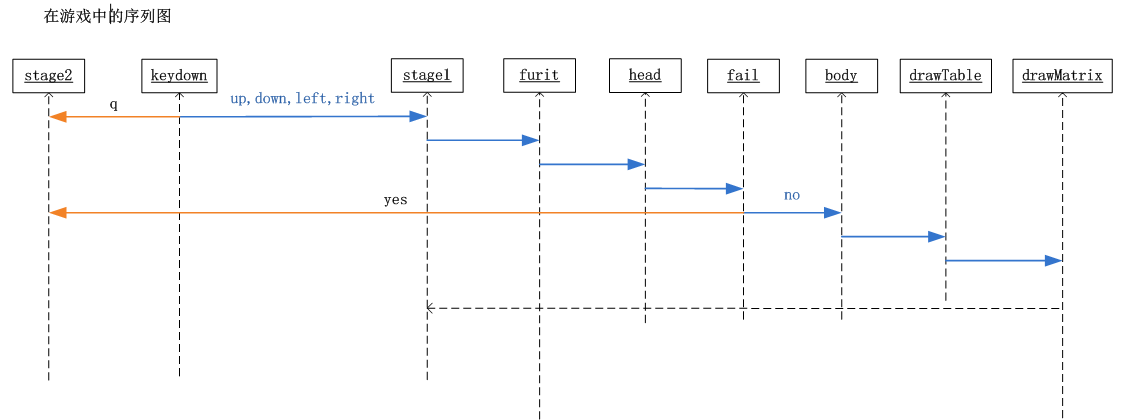## 2. 游戏框架定义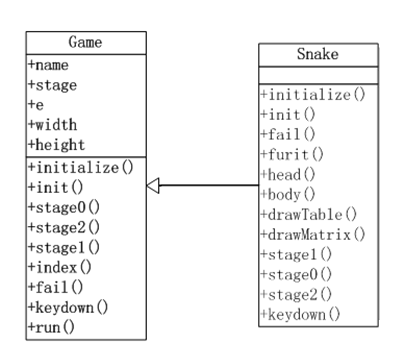• Game类公共属性，包括了所有的Snake类的属性，这是因为这些属性都是全局的，其他的游戏也会用到，而且每个游戏中的属性，可以在e中进行定义。
• Game类公共方法，包括了游戏全局调用的方法，但不包括Snake游戏stage1场景中运行的方法。在Game类的方法中，我们主要实现的都是开发的辅助功能。
• Snake类方法，同样还有Game类的方法，这是用到方法的重写技术。子类的方法，先调用父类的同名方法，然后再执行子类方法里的程序。

## 3. 在框架中重新实现贪食蛇游戏

Game类的代码实现，用R语言的RC面向对象编程进行代码编写。

``````
Game<-setRefClass('Game',

fields=list(
# 系统变量
name="character", # 名字
debug='logical',  # 调试状态
width='numeric',  # 矩阵宽
height='numeric', # 矩阵高

# 应用变量
stage='numeric',  # 场景
e='environment',  # 环境空间变量
m='matrix',       # 数据矩阵
isFail='logical'  # 游戏失败
),

methods=list(

# 构造函数
initialize = function(name,width,height,debug) {
name<<-"R Game Framework"
debug<<-FALSE
width<<-height<<-20   #矩阵宽高
},

# 初始化变量
init = function(){
e<<-new.env()   #环境空间
m<<-matrix(rep(0,width*height),nrow=width)  #数据矩阵
isFail<<-FALSE
},

# 开机画图
stage0=function(){
stage<<-0
init()
},

# 结束画图
stage2=function(){
stage<<-2
},

# 游戏中
stage1=function(default=FALSE){
stage<<-1
if(FALSE){  # 默认游戏中界面
plot(0,0,xlim=c(0,1),ylim=c(0,1),type='n',xaxs="i", yaxs="i")
text(0.5,0.7,label="Playing",cex=5)
}
},

# 矩阵工具
index = function(col) {
return(which(m==col))
},

# 失败操作
fail=function(msg){
print(paste("Game Over",msg))
isFail<<-TRUE
keydown('q')
return(NULL)
},

# 键盘事件，控制场景切换
keydown=function(K){
if(stage==0){ #开机画面
stage1()
return(NULL)
}

if(stage==2){ #结束画面
if(K=="q") q()
else if(K==' ') stage0()
return(NULL)
}
},

# 启动程序
run=function(){
par(mai=rep(0,4),oma=rep(0,4))
stage0()
getGraphicsEvent(prompt="Snake Game",onKeybd=function(K){
if(debug) print(paste("keydown",K))
return(keydown(K))
})
}
)
)
``````

Snake类的代码实现，继承Game类，并实现贪食蛇游戏的私有方法。

``````
# 引用game.r文件
source(file="game.r")

# Snake类，继承Game类
Snake<-setRefClass("Snake",contains="Game",

methods=list(

# 构造函数
initialize = function(name,width,height,debug) {
callSuper(name,width,height,debug) # 调父类

name<<-"Snake Game"
},

# 初始化变量
init = function(){
callSuper()  # 调父类

e\$step<<-1/width #步长
e\$dir<<-e\$lastd<<-'up' # 移动方向
e\$lastx<<-e\$lasty<<-2 # 蛇头上一个点坐标
e\$tail<<-data.frame(x=c(),y=c())#初始蛇尾坐标

e\$col_furit<<-2 #水果颜色
e\$col_tail<<-8 #蛇尾颜色
e\$col_path<<-0 #路颜色
e\$col_barrier<<-1 #障碍颜色
},

# 失败检查
lose=function(){
fail("Out of ledge.")
return(NULL)
}

return(NULL)
}
},

# 随机的水果点
furit=function(){
if(length(index(e\$col_furit))<=0){ #不存在水果
idx<-sample(index(e\$col_path),1)

fx<-ifelse(idx%%width==0,10,idx%%width)
fy<-ceiling(idx/height)
m[fx,fy]<<-e\$col_furit

if(debug){
print(paste("furit idx",idx))
print(paste("furit axis:",fx,fy))
}
}
},

# 方向操作
},

# snake body
body=function(){
if(isFail) return(NULL)

m[e\$lastx,e\$lasty]<<-e\$col_path
if(length(index(e\$col_furit))<=0){ #不存在水果
e\$tail<<-rbind(e\$tail,data.frame(x=e\$lastx,y=e\$lasty))
}

if(nrow(e\$tail)>0) { #如果有尾巴
e\$tail<<-rbind(e\$tail,data.frame(x=e\$lastx,y=e\$lasty))
m[e\$tail[1,]\$x,e\$tail[1,]\$y]<<-e\$col_path
e\$tail<<-e\$tail[-1,]
m[e\$lastx,e\$lasty]<<-e\$col_tail
}

if(debug){
}
},

# 画布背景
drawTable=function(){
if(isFail) return(NULL)

plot(0,0,xlim=c(0,1),ylim=c(0,1),type='n',xaxs="i", yaxs="i")

if(debug){
# 显示背景表格
abline(h=seq(0,1,e\$step),col="gray60") # 水平线
abline(v=seq(0,1,e\$step),col="gray60") # 垂直线
# 显示矩阵
df<-data.frame(x=rep(seq(0,0.95,e\$step),width),y=rep(seq(0,0.95,e\$step),each=height),lab=seq(1,width*height))
text(df\$x+e\$step/2,df\$y+e\$step/2,label=df\$lab)
}
},

# 根据矩阵画数据
drawMatrix=function(){
if(isFail) return(NULL)

idx<-which(m>0)
px<- (ifelse(idx%%width==0,width,idx%%width)-1)/width+e\$step/2
py<- (ceiling(idx/height)-1)/height+e\$step/2
pxy<-data.frame(x=px,y=py,col=m[idx])
points(pxy\$x,pxy\$y,col=pxy\$col,pch=15,cex=4.4)
},

# 游戏场景
stage1=function(){
callSuper()

furit()
lose()
body()
drawTable()
drawMatrix()
},

# 开机画图
stage0=function(){
callSuper()
plot(0,0,xlim=c(0,1),ylim=c(0,1),type='n',xaxs="i", yaxs="i")
text(0.5,0.7,label=name,cex=5)
text(0.5,0.4,label="Any keyboard to start",cex=2,col=4)
text(0.5,0.3,label="Up,Down,Left,Rigth to control direction",cex=2,col=2)
text(0.2,0.05,label="Author:DanZhang",cex=1)
text(0.5,0.05,label="http://blog.fens.me",cex=1)
},

# 结束画图
stage2=function(){
callSuper()
info<-paste("Congratulations! You have eat",nrow(e\$tail),"fruits!")
print(info)

plot(0,0,xlim=c(0,1),ylim=c(0,1),type='n',xaxs="i", yaxs="i")
text(0.5,0.7,label="Game Over",cex=5)
text(0.5,0.4,label="Space to restart, q to quit.",cex=2,col=4)
text(0.5,0.3,label=info,cex=2,col=2)
text(0.2,0.05,label="Author:DanZhang",cex=1)
text(0.5,0.05,label="http://blog.fens.me",cex=1)
},

# 键盘事件，控制场景切换
keydown=function(K){
callSuper(K)

if(stage==1){ #游戏中
if(K == "q") stage2()
else {
if(tolower(K) %in% c("up","down","left","right")){
e\$lastd<<-e\$dir
e\$dir<<-tolower(K)
stage1()
}
}
return(NULL)
}
return(NULL)
}
)
)

snake<-function(){
game<-Snake\$new()
game\$initFields(debug=TRUE)
game\$run()
}

snake()
``````## R语言基于RC的面向对象编程

R的极客理想系列文章，涵盖了R的思想，使用，工具，创新等的一系列要点，以我个人的学习和体验去诠释R的强大。

R语言作为统计学一门语言，一直在小众领域闪耀着光芒。直到大数据的爆发，R语言变成了一门炙手可热的数据分析的利器。随着越来越多的工程背景的人的加入，R语言的社区在迅速扩大成长。现在已不仅仅是统计领域，教育，银行，电商，互联网….都在使用R语言。

• 张丹(Conan), 程序员Java,R,PHP,Javascript
• weibo：@Conan_Z
• blog: http://blog.fens.me
• email: bsspirit@gmail.com

http://blog.fens.me/r-class-rc/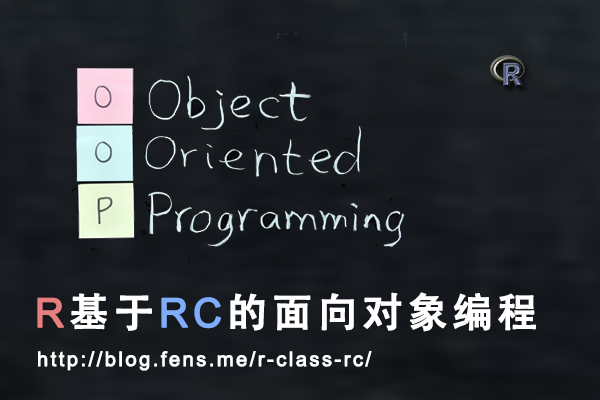RC对象系统从底层上改变了原有S3和S4对象系统的设计，去掉了泛型函数，真正地以类为基础实现面向对象的特征。

1. RC对象系统介绍
2. 创建RC类和对象
3. 对象赋值
4. 定义对象的方法
5. RC对象内置方法
6. RC类的辅助构造函数
7. RC对象系统的使用

## 1 RC对象系统介绍

RC是Reference classes的简称，又被称为R5，在R语言的2.12版本被引入的，是最新一代的面向对象系统。

RC不同于原来的S3和S4对象系统，RC对象系统的方法是在类中自定的，而不是泛型函数。RC对象的行为更相似于其他的编程语言，实例化对象的语法也有所改变。

• 类：面向对象系统的基本类型，类是静态结构定义。
• 对象：类实例化后，在内存中生成结构体。
• 方法：是类中的函数定义，不通过泛型函数实现。

## 2 创建RC类和对象

• Linux: Ubuntu Server 12.04.2 LTS 64bit
• R: 3.0.1 x86_64-pc-linux-gnu

``````
# 加载pryr包
> library(pryr)
``````

2.1 如何创建RC类？

RC对象系统是以类为基本类型， 有专门的类的定义函数 setRefClass() 和 实例化则通过类的方法生成，我们一下如何用RC对象系统创建一个类。

2.1.1 setRefClass()

``````
setRefClass(Class, fields = , contains = , methods =, where =, ...)
``````

• Class: 定义类名
• fields: 定义属性和属性类型
• contains: 定义父类，继承关系
• methods: 定义类中的方法
• where: 定义存储空间

2.2 创建RC类和实例

``````
# 定义一个RC类
> User<-setRefClass("User",fields=list(name="character"))

# 查看User的定义
> User
Generator for class "User":

Class fields:
Name:       name
Class: character

Class Methods:
"callSuper", "copy", "export", "field", "getClass",
"getRefClass", "import", "initFields", "show", "trace",
"untrace", "usingMethods"

Reference Superclasses:
"envRefClass"

# 实例化一个User对象u1
> u1<-User\$new(name="u1")

# 查看u1对象
> u1
Reference class object of class "User"
Field "name":
 "u1"

# 检查User类的类型
> class(User)
 "refObjectGenerator"
attr(,"package")
 "methods"
> is.object(User)
 TRUE
> otype(User)
 "RC"

# 检查u1的类型
> class(u1)
 "User"
attr(,"package")
 ".GlobalEnv"
> is.object(u1)
 TRUE
> otype(u1)
 "RC"
``````

2.3 创建一个有继承关系的RC类

``````
# 创建RC类User
> User<-setRefClass("User",fields=list(name="character"))

# 创建User的子类Member
> Member<-setRefClass("Member",contains="User",fields=list(manager="User"))

# 实例化User
> manager<-User\$new(name="manager")

# 实例化一个Son对象
> member<-Member\$new(name="member",manager=manager)

# 查看member对象
> member
Reference class object of class "Member"
Field "name":
 "member"
Field "manager":
Reference class object of class "User"
Field "name":
 "manager"

# 查看member对象的name属性
> member\$name
 "member

# 查看member对象的manager属性
> member\$manager
Reference class object of class "User"
Field "name":
 "manager"

# 检查对象的属性类型
> otype(member\$name)
 "primitive"
> otype(member\$manager)
 "RC"
``````

2.4 RC对象的默认值

RC的类有一个指定构造器方法 \$initialize()，这个构造器方法在实例化对象时，会自动被运行一次，通过这个构造方法可以设置属性的默认值。

``````
# 定义一个RC类
> User<-setRefClass("User",
+
+     # 定义2个属性
+     fields=list(name="character",level='numeric'),
+     methods=list(
+
+          # 构造方法
+          initialize = function(name,level){
+              print("User::initialize")
+
+              # 给属性增加默认值
+              name <<- 'conan'
+              level <<- 1
+           }
+      )
+ )

# 实例化对象u1
> u1<-User\$new()
 "User::initialize"

# 查看对象u1，属性被增加了默认值
> u1
Reference class object of class "User"
Field "name":
 "conan"
Field "level":
 1
``````

## 3 对象赋值

``````
# 定义User类
> User<-setRefClass("User",fields=list(name="character",age="numeric",gender="factor"))

# 定义一个factor类型
> genderFactor<-factor(c('F','M'))

# 实例化u1
> u1<-User\$new(name="u1",age=44,gender=genderFactor)

# 查看age属性值
> u1\$age
 44
``````

``````
# 重新赋值
> u1\$age<-10

# age属性改变
> u1\$age
 10
``````

``````
# 把u1赋值给u2对象
> u2<-u1

# 查看u2的age属性
> u2\$age
 10

# 重新赋值
> u1\$age<-20

# 查看u1,u2的age属性，都发生改变
> u1\$age
 20
> u2\$age
 20
``````

``````
# 调用u1的内置方法copy()，赋值给u3
> u3<-u1\$copy()

# 查看u3的age属性
> u3\$age
 20

# 重新赋值
> u1\$age<-30

# 查看u1的age属性，发生改变
> u1\$age
 30

# 查看u3的age属性，没有改变
> u3\$age
 20
``````

## 4 定义对象的方法

``````
# 定义一个RC类，包括方法
> User<-setRefClass("User",
+       fields=list(name="character",favorite="vector"),
+
+       # 方法属性
+       methods = list(
+
+           # 增加一个兴趣
+                 favorite <<- c(favorite,x)
+           },
+
+           # 删除一个兴趣
+           delFavorite = function(x) {
+                 favorite <<- favorite[-which(favorite == x)]
+           },
+
+           # 重新定义兴趣列表
+           setFavorite = function(x) {
+                 favorite <<- x
+           }
+       )
+ )

# 实例化对象u1
> u1<-User\$new(name="u1",favorite=c('movie','football'))

# 查看u1对象
> u1
Reference class object of class "User"
Field "name":
 "u1"
Field "favorite":
 "movie"    "football"
``````

``````
# 删除一个兴趣
> u1\$delFavorite('football')

# 查看兴趣属性
> u1\$favorite
 "movie"

# 增加一个兴趣
> u1\$favorite
 "movie"    "shopping"

# 重置兴趣列表
> u1\$favorite
``````

## 5 RC对象内置方法和内置属性

5.1 内置方法：

• initialize 类的初始化函数，用于设置属性的默认值，只能在类定义的方法中使用。
• callSuper 调用父类的同名方法，只能在类定义的方法中使用。
• copy 复制实例化对象的所有属性。
• initFields 给对象的属性赋值。
• field 查看属性或给属性赋值。
• getClass 查看对象的类定义。
• getRefClass 同getClass。
• show 查看当前对象。
• export 查看属性值以类为作用域。
• import 把一个对象的属性值赋值给另一个对象。
• trace 跟踪对象中方法调用，用于程序debug。
• untrace 取消跟踪。
• usingMethods 用于实现方法调用，只能在类定义的方法中使用。这个方法不利于程序的健壮性，所以不建议使用。

``````
# 类User
> User<-setRefClass("User",
+    fields=list(name="character",level='numeric'),
+    methods=list(
+      initialize = function(name,level){
+        print("User::initialize")
+        name <<- 'conan'
+        level <<- 1
+      },
+        level <<- level+x
+      },
+      }
+    )
+)
``````

``````
# 子类Member
> Member<-setRefClass("Member",contains="User",
+
+    # 子类中的属性
+    fields=list(age='numeric'),
+    methods=list(
+
+      # 覆盖父类的同名方法
+
+          # 调用父类的同名方法
+          callSuper(x)
+          level <<- level+1
+      }
+    )
+)
``````

``````
# 实例化u1
> u1<-User\$new(name='u1',level=10)
 "User::initialize"

# 查看u1对象，\$new()不能实现赋值的操作
> u1
Reference class object of class "User"
Field "name":
 "conan"
Field "level":
 1

# 通过\$initFields()向属性赋值
> u1\$initFields(name='u1',level=10)
Reference class object of class "User"
Field "name":
 "u1"
Field "level":
 10

# 实例化m1
> m1<-Member\$new()
 "User::initialize"

> m1\$initFields(name='m1',level=100,age=12)
Reference class object of class "Member"
Field "name":
 "m1"
Field "level":
 100
Field "age":
 12
``````

``````
# 属性复制到u2
> u2<-u1\$copy()
 "User::initialize"

Reference class object of class "User"
Field "name":
 "u1"
Field "level":
 11

# u2的level与u1没有引入关系，u2没有变化
> u2
Reference class object of class "User"
Field "name":
 "u1"
Field "level":
 10
``````

``````
# 查看level属性值
> u1\$field('level')
 11

# 给level赋值为1
> u1\$field('level',1)

# 查看level属性值
> u1\$level
 1
``````

``````
# 类引入的定义
> m1\$getRefClass()
Generator for class "Member":

Class fields:
Name:       name     level       age
Class: character   numeric   numeric

Class Methods:
"initialize", "show", "trace", "untrace", "usingMethods"

Reference Superclasses:
"User", "envRefClass"

# 类定义
> m1\$getClass()
Reference Class "Member":

Class fields:
Name:       name     level       age
Class: character   numeric   numeric

Class Methods:
"initialize", "show", "trace", "untrace", "usingMethods"

Reference Superclasses:
"User", "envRefClass"

# 通过otype查看类型的不同
> otype(m1\$getRefClass())
 "RC"
> otype(m1\$getClass())
 "S4"
``````

``````
> m1\$show()
Reference class object of class "Member"
Field "name":
 "m1"
Field "level":
 100
Field "age":
 12

> show(m1)
Reference class object of class "Member"
Field "name":
 "m1"
Field "level":
 100
Field "age":
 12

> m1
Reference class object of class "Member"
Field "name":
 "m1"
Field "level":
 100
Field "age":
 12
``````

``````
Tracing reference method "addLevel" for object from class "Member"

Untracing reference method "addLevel" for object from class "Member"
``````

``````
# 查看在Member类中的属性
> m1\$export('Member')
Reference class object of class "Member"
Field "name":
 "m1"
Field "level":
 105
Field "age":
 12

# 查看在User类中的属性，当前作用域不包括age属性。
> m1\$export('User')
 "User::initialize"
Reference class object of class "User"
Field "name":
 "m1"
Field "level":
 105
``````

``````
# 实例化m2
> m2<-Member\$new()
 "User::initialize"
> m2
Reference class object of class "Member"
Field "name":
 "conan"
Field "level":
 1
Field "age":
numeric(0)

# 把m1对象的值赋值给m2对象
> m2\$import(m1)
> m2
Reference class object of class "Member"
Field "name":
 "m1"
Field "level":
 105
Field "age":
 12
``````

5.2 内置属性：

RC对象实例化后，有两个内置属性：

• .self 实例化对象自身
• .refClassDef 类的定义类型
``````
# \$.self属性
> m1\$.self
Reference class object of class "Member"
Field "name":
 "m1"
Field "level":
 105
Field "age":
 12

#  m1\$.self和m1 完全相同
> identical(m1\$.self,m1)
 TRUE

# 查看类型
> otype(m1\$.self)
 "RC"

# \$.refClassDef属性
> m1\$.refClassDef
Reference Class "Member":

Class fields:
Name:       name     level       age
Class: character   numeric   numeric

Class Methods:
"initialize", "show", "trace", "untrace", "usingMethods"

Reference Superclasses:
"User", "envRefClass"

# 与\$getClass()相同
> identical(m1\$.refClassDef,m1\$getClass())
 TRUE

# 查看类型
> otype(m1\$.refClassDef)
 "S4"
``````

## 6 RC类的辅助函数

• new 用于实例化对象。
• help 用于查询类中方法的调用。
• methods 列出类中定义的所有方法。
• fields 列出类中定义的所有属性。
• lock 给属性加锁，实例化对象的属性只允许赋值一次，即赋值变量，不可修改。
• trace 跟踪方法。
• accessors 给属性生成get/set方法。

``````
# 定义User类
> User<-setRefClass("User",
+    fields=list(name="character",level='numeric'),
+    methods=list(
+      initialize = function(name,level){
+        print("User::initialize")
+        name <<- 'conan'
+        level <<- 1
+      },
+        level <<- level+x
+      },
+      }
+    )
+)

# 实例化对象u1
> u1<-User\$new()

# 列出User类中的属性
> User\$fields()
name       level
"character"   "numeric"

# 列出User类中的方法
> User\$methods()
 "copy"         "export"       "field"
 "getClass"     "getRefClass"  "import"
 "initFields"   "initialize"   "show"
 "trace"        "untrace"      "usingMethods"

# 查看User类的方法调用
Call:

> User\$help("show")
Call:
\$show()
``````

``````
# 给level属性增加get/set方法
> User\$accessors("level")

# 给name属性增加get/set方法
> User\$accessors("name")

# 列出所有方法
> User\$methods()
 "copy"         "export"       "field"
 "getClass"     "getLevel"     "getName"
 "getRefClass"  "import"       "initFields"
 "initialize"   "setLevel"     "setName"
 "show"         "trace"        "untrace"
 "usingMethods"
``````

``````
Tracing reference method "addLevel" for class
"User"

# 实例化对象u3
> u3<-User\$new(name='u3',level=1)
 "User::initialize"

``````

``````
# 锁定level属性
> User\$lock("level")

# 查看User类中被锁定的属性
> User\$lock()
 "level"

# 实例化对象u3，这时level属性已经被赋值一次
> u3<-User\$new()
 "User::initialize"
> u3
Reference class object of class "User"
Field "name":
 "conan"
Field "level":
 1

# 给level属性，再次赋值出错
> u3\$level=1
Error: invalid replacement: reference class field ‘level’ is read-only
Error: invalid replacement: reference class field ‘level’ is read-only
``````

## 7 RC对象系统的使用

7.1 任务一：定义动物的数据结构和发声方法。

• 定义动物的数据结构
• 分别定义3种动物的发声bark()方法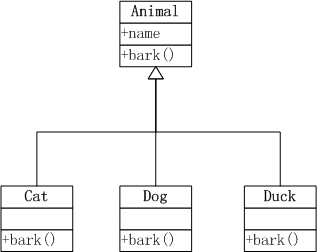``````
# 创建Animal类，包括name属性，构造方法initialize()，叫声方法bark()。
> Animal<-setRefClass("Animal",
+  fields=list(name="character"),
+  methods=list(
+    initialize = function(name) name <<- 'Animal',
+    bark = function() print("Animal::bark")
+  )
+)

# 创建Cat类，继承Animal类，并重写(Overwrite)了 initialize() 和 bark()。
> Cat<-setRefClass("Cat",contains="Animal",
+  methods=list(
+    initialize = function(name) name <<- 'cat',
+    bark = function() print(paste(name,"is miao miao"))
+  )
+)

# 创建Dog类，继承Animal类，并重写(Overwrite)了 initialize() 和 bark()。
> Dog<-setRefClass("Dog",contains="Animal",
+  methods=list(
+    initialize = function(name) name <<- 'dog',
+    bark = function() print(paste(name,"is wang wang"))
+  )
+)

# 创建Duck类，继承Animal类，并重写(Overwrite)了 initialize() 和 bark()。
> Duck<-setRefClass("Duck",contains="Animal",
+   methods=list(
+     initialize = function(name) name <<- 'duck',
+     bark = function() print(paste(name,"is ga ga"))
+   )
+)
``````

``````
# 创建cat实例
> cat<-Cat\$new()
> cat\$name
 "cat"

# cat叫声
> cat\$bark()
 "cat is miao miao"

# 创建dog实例，并给dog起名叫Huang
> dog<-Dog\$new()
> dog\$initFields(name='Huang')
Reference class object of class "Dog"
Field "name":
 "Huang"
> dog\$name
 "Huang"

# dog叫声
> dog\$bark()
 "Huang is wang wang"

# 创建duck实例
> duck<-Duck\$new()

# duck叫声
> duck\$bark()
 "duck is ga ga"
``````

7.2 任务二：定义动物的体貌特征

3种动物都有肢，cat和dog是四肢，duck是二肢和二翅。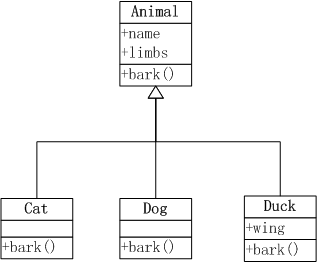``````
# 定义Animal类，增加limbs属性，默认值为4
Animal<-setRefClass("Animal",
fields=list(name="character",limbs='numeric'),
methods=list(
initialize = function(name) {
name <<- 'Animal'
limbs<<-4
},
bark = function() print("Animal::bark")
)
)

# 在Cat类的 initialize()方法中，执行callSuper()方法，调用父类的同名方法
Cat<-setRefClass("Cat",contains="Animal",
methods=list(
initialize = function(name) {
callSuper()
name <<- 'cat'
},
bark = function() print(paste(name,"is miao miao"))
)
)

# 在Dog类的 initialize()方法中，执行callSuper()方法，调用父类的同名方法
Dog<-setRefClass("Dog",contains="Animal",
methods=list(
initialize = function(name) {
callSuper()
name <<- 'dog'
},
bark = function() print(paste(name,"is wang wang"))
)
)

# 在Dog类的定义wing属性， 并在initialize()方法，定义limbs和wing属性的默认值
Duck<-setRefClass("Duck",contains="Animal",
fields=list(wing='numeric'),
methods=list(
initialize = function(name) {
name <<- 'duck'
limbs<<- 2
wing<<- 2
},
bark = function() print(paste(name,"is ga ga"))
)
)
``````

``````
# 实例化cat对象，属性limbs为4
> cat<-Cat\$new();cat
Reference class object of class "Cat"
Field "name":
 "cat"
Field "limbs":
 4

# 实例化dog对象，属性limbs为4
> dog<-Dog\$new()
> dog\$initFields(name='Huang')
Reference class object of class "Dog"
Field "name":
 "Huang"
Field "limbs":
 4
> dog
Reference class object of class "Dog"
Field "name":
 "Huang"
Field "limbs":
 4

# 实例化duck对象，属性limbs为2，wing为2
> duck<-Duck\$new();duck
Reference class object of class "Duck"
Field "name":
 "duck"
Field "limbs":
 2
Field "wing":
 2
``````

7.3 任务三：定义动物的行动方式。

• 猫(cat) 爬树
• 狗(dog) 游泳
• 鸭(duck) 游泳，短距离飞行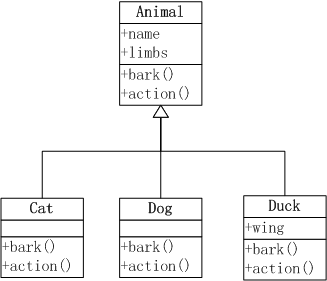``````
# 定义类Animal，增加action()方法，用于通用的行为陆地上行动。
> Animal<-setRefClass("Animal",
+    fields=list(name="character",limbs='numeric'),
+    methods=list(
+      initialize = function(name) {
+        name <<- 'Animal'
+        limbs<<-4
+      },
+      bark = function() print("Animal::bark"),
+      action = function() print("I can walk on the foot")
+    )
+)

# 定义Cat类，重写action()方法，并增加爬树的行动
> Cat<-setRefClass("Cat",contains="Animal",
+     methods=list(
+       initialize = function(name) {
+         callSuper()
+         name <<- 'cat'
+       },
+       bark = function() print(paste(name,"is miao miao")),
+       action = function() {
+         callSuper()
+         print("I can Climb a tree")
+       }
+     )
+  )

# 定义Dog类，重写action()方法，并增加游泳行动
> Dog<-setRefClass("Dog",contains="Animal",
+   methods=list(
+     initialize = function(name) {
+       callSuper()
+       name <<- 'dog'
+     },
+     bark = function() print(paste(name,"is wang wang")),
+     action = function() {
+         callSuper()
+         print("I can Swim.")
+     }
+   )
+)

# 定义Duck类，重写action()方法，并增加游泳和短距离飞行
> Duck<-setRefClass("Duck",contains="Animal",
+    fields=list(wing='numeric'),
+    methods=list(
+      initialize = function(name) {
+        name <<- 'duck'
+        limbs<<- 2
+        wing<<- 2
+      },
+      bark = function() print(paste(name,"is ga ga")),
+      action = function() {
+        callSuper()
+        print("I can swim.")
+        print("I also can fly a short way.")
+     }
+    )
+)
``````

cat的行动。

``````
# 实例化cat
> cat<-Cat\$new()

# cat的行动
> cat\$action()
 "I can walk on the foot"
 "I can Climb a tree"
``````

dog的行动。

``````
> dog<-Dog\$new()
> dog\$action()
 "I can walk on the foot"
 "I can Swim."
``````

duck的行动。

``````
> duck<-Duck\$new()
> duck\$action()
 "I can walk on the foot"
 "I can swim."
 "I also can fly a short way."
``````

http://blog.fens.me/r-class-rc/## R语言基于S4的面向对象编程

R的极客理想系列文章，涵盖了R的思想，使用，工具，创新等的一系列要点，以我个人的学习和体验去诠释R的强大。

R语言作为统计学一门语言，一直在小众领域闪耀着光芒。直到大数据的爆发，R语言变成了一门炙手可热的数据分析的利器。随着越来越多的工程背景的人的加入，R语言的社区在迅速扩大成长。现在已不仅仅是统计领域，教育，银行，电商，互联网….都在使用R语言。

• 张丹(Conan), 程序员Java,R,PHP,Javascript
• weibo：@Conan_Z
• blog: http://blog.fens.me
• email: bsspirit@gmail.com

http://blog.fens.me/r-class-s4/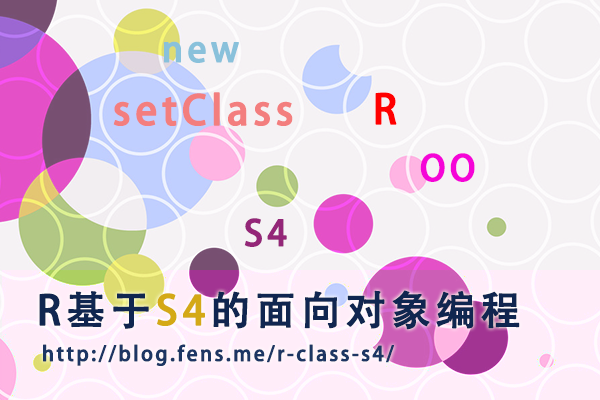S4对象系统具有明显的结构化特征，更适合面向对象的程序设计。Bioconductor社区，以S4对象系统做为基础架构，只接受符合S4定义的R包。

1. S4对象介绍
2. 创建S4对象
3. 访问对象的属性
4. S4的泛型函数
5. 查看S4对象的函数
6. S4对象的使用

## 1 S4对象介绍

S4对象系统是一种标准的R语言面向对象实现方式，S4对象有明确的类定义，参数定义，参数检查，继承关系，实例化等的面向对象系统的特征。

## 2 创建S4对象

• Linux: Ubuntu Server 12.04.2 LTS 64bit
• R: 3.0.1 x86_64-pc-linux-gnu

``````
# 加载pryr包
> library(pryr)
``````

2.1 如何创建S4对象？

2.1.1 setClass()

``````
setClass(Class, representation, prototype, contains=character(),
validity, access, where, version, sealed, package,
S3methods = FALSE, slots)
``````

• Class: 定义类名
• slots: 定义属性和属性类型
• prototype: 定义属性的默认值
• contains=character(): 定义父类，继承关系
• validity: 定义属性的类型检查
• where: 定义存储空间
• sealed: 如果设置TRUE，则同名类不能被再次定义
• package: 定义所属的包
• S3methods: R3.0.0以后不建议使用
• representation R3.0.0以后不建议使用
• access R3.0.0以后不建议使用
• version R3.0.0以后不建议使用

2.2 创建一个S4对象实例

``````
# 定义一个S4对象
> setClass("Person",slots=list(name="character",age="numeric"))

# 实例化一个Person对象
> father<-new("Person",name="F",age=44)

# 查看father对象，有两个属性name和age
> father
An object of class "Person"
Slot "name":
 "F"

Slot "age":
 44

# 查看father对象类型，为Person
> class(father)
 "Person"
attr(,"package")
 ".GlobalEnv"

# 查看father对象为S4的对象
> otype(father)
 "S4"
``````

2.3 创建一个有继承关系的S4对象

``````
# 创建一个S4对象Person
> setClass("Person",slots=list(name="character",age="numeric"))

# 创建Person的子类
> setClass("Son",slots=list(father="Person",mother="Person"),contains="Person")

# 实例化Person对象
> father<-new("Person",name="F",age=44)
> mother<-new("Person",name="M",age=39)

# 实例化一个Son对象
> son<-new("Son",name="S",age=16,father=father,mother=mother)

# 查看son对象的name属性
> son@name
 "S"

# 查看son对象的age属性
> son@age
 16

# 查看son对象的father属性
> son@father
An object of class "Person"
Slot "name":
 "F"

Slot "age":
 44

# 查看son对象的mother属性
> slot(son,"mother")
An object of class "Person"
Slot "name":
 "M"

Slot "age":
 39

# 检查son类型
> otype(son)
 "S4"

# 检查son@name属性类型
> otype(son@name)
 "primitive"

# 检查son@mother属性类型
> otype(son@mother)
 "S4"

# 用isS4()，检查S4对象的类型
> isS4(son)
 TRUE
> isS4(son@name)
 FALSE
> isS4(son@mother)
 TRUE
``````

2.4 S4对象的默认值

``````
> setClass("Person",slots=list(name="character",age="numeric"))

# 属性age为空
> a<-new("Person",name="a")
> a
An object of class "Person"
Slot "name":
 "a"

Slot "age":
numeric(0)

# 设置属性age的默认值20
> setClass("Person",slots=list(name="character",age="numeric"),prototype = list(age = 20))

# 属性age为空
> b<-new("Person",name="b")

# 属性age的默认值是20
> b
An object of class "Person"
Slot "name":
 "b"

Slot "age":
 20
``````

2.5 S4对象的类型检查

``````
> setClass("Person",slots=list(name="character",age="numeric"))

# 传入错误的age类型
Error in validObject(.Object) :
invalid class “Person” object: invalid object for slot "age" in class "Person": got class "character", should be or extend class "numeric"

# 设置age的非负检查
> setValidity("Person",function(object) {
+     if (object@age <= 0) stop("Age is negative.")
+ })
Class "Person" [in ".GlobalEnv"]

Slots:
Name:       name       age
Class: character   numeric

# 修传入小于0的年龄
Error in validityMethod(object) : Age is negative.
``````

2.6 从一个已经实例化的对象中创建新对象

S4对象，还支持从一个已经实例化的对象中创建新对象，创建时可以覆盖旧对象的值

``````
> setClass("Person",slots=list(name="character",age="numeric"))

# 创建一个对象实例n1
> n1<-new("Person",name="n1",age=19);n1
An object of class "Person"
Slot "name":
 "n1"

Slot "age":
 19

# 从实例n1中，创建实例n2，并修改name的属性值
> n2<-initialize(n1,name="n2");n2
An object of class "Person"
Slot "name":
 "n2"

Slot "age":
 19
``````

## 3 访问对象的属性

``````
> setClass("Person",slots=list(name="character",age="numeric"))
> a<-new("Person",name="a")

# 访问S4对象的属性
> a@name
 "a"
> slot(a, "name")
 "a"

# 错误的属性访问
> a\$name
Error in a\$name : \$ operator not defined for this S4 class
> a
Error in a : object of type 'S4' is not subsettable
> a[]
Error in a[] : this S4 class is not subsettable
``````

## 4 S4的泛型函数

S4的泛型函数实现有别于S3的实现，S4分离了方法的定义和实现，如在其他语言中我们常说的接口和实现分离。通过setGeneric()来定义接口，通过setMethod()来定义现实类。这样可以让S4对象系统，更符合面向对象的特征。

``````
> work<-function(x) cat(x, "is working")
> work('Conan')
Conan is working
``````

``````
# 定义Person对象
> setClass("Person",slots=list(name="character",age="numeric"))

# 定义泛型函数work，即接口
> setGeneric("work",function(object) standardGeneric("work"))
 "work"

# 定义work的现实，并指定参数类型为Person对象
> setMethod("work", signature(object = "Person"), function(object) cat(object@name , "is working") )
 "work"

# 创建一个Person对象a
> a<-new("Person",name="Conan",age=16)

# 把对象a传入work函数
> work(a)
Conan is working
``````

• 定义数据对象类型
• 定义接口函数
• 定义实现函数
• 把数据对象以参数传入到接口函数，执行实现函数

## 5 查看S4对象的函数

``````
# 检查work的类型
> ftype(work)
 "s4"      "generic"

# 直接查看work函数
> work
standardGeneric for "work" defined from package ".GlobalEnv"
function (object)
standardGeneric("work")
<environment: 0x2aa6b18>
Methods may be defined for arguments: object
Use  showMethods("work")  for currently available ones.

# 查看work函数的现实定义
> showMethods(work)
Function: work (package .GlobalEnv)
object="Person"

# 查看Person对象的work函数现实
> getMethod("work", "Person")
Method Definition:
function (object)
cat(object@name, "is working")
Signatures:
object
target  "Person"
defined "Person"

> selectMethod("work", "Person")
Method Definition:
function (object)
cat(object@name, "is working")
Signatures:
object
target  "Person"
defined "Person"

# 检查Person对象有没有work函数
>  existsMethod("work", "Person")
 TRUE
> hasMethod("work", "Person")
 TRUE
``````

## 6 S4对象的使用

6.1 任务一：定义图形库的数据结构和计算函数

• 定义图形库的数据结构
• 定义圆形的数据结构，并计算面积和周长
• 定义椭圆形的数据结构，并计算面积和周长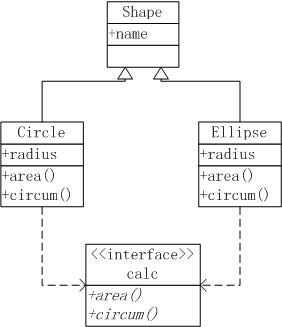``````
# 定义基类Shape
> setClass("Shape",slots=list(name="character"))

> setValidity("Circle",function(object) {
+ })
Class "Circle" [in ".GlobalEnv"]
Slots:
Class:   numeric character
Extends: "Shape"

# 创建两个圆形实例
> c1<-new("Circle",name="c1")
``````

``````
# 计算面积泛型函数接口
> setGeneric("area",function(obj,...) standardGeneric("area"))
 "area"

# 计算面积的函数现实
> setMethod("area","Circle",function(obj,...){
+     print("Area Circle Method")
+ })
 "area"

# 分别计算c1和c2的两个圆形的面积
> area(c1)
 "Area Circle Method"
 3.141593
> area(c2)
 "Area Circle Method"
 78.53982
``````

``````
# 计算周长泛型函数接口
> setGeneric("circum",function(obj,...) standardGeneric("circum"))
 "circum"

# 计算周长的函数现实
> setMethod("circum","Circle",function(obj,...){
+ })

# 分别计算c1和c2的两个圆形的面积
 "circum"
> circum(c1)
 6.283185
> circum(c2)
 31.41593
``````

``````

> setValidity("Ellipse",function(object) {
+     if (length(object@radius) != 2 ) stop("It's not Ellipse.")
+ })
Class "Ellipse" [in ".GlobalEnv"]
Slots:
Class:   numeric character
Extends: "Shape"

# 创建两个椭圆形实例e1,e2
> e1<-new("Ellipse",name="e1")

# 计算椭圆形面积的函数现实
> setMethod("area", "Ellipse",function(obj,...){
+     print("Area Ellipse Method")
+ })
 "area"

# 计算e1,e2两个椭圆形的面积
> area(e1)
 "Area Ellipse Method"
 3.141593
> area(e2)
 "Area Ellipse Method"
 15.70796

# 计算椭圆形周长的函数现实
> setMethod("circum","Ellipse",function(obj,...){
+     cat("Ellipse Circum :\n")
+ })
 "circum"

# 计算e1,e2两个椭圆形的周长
> circum(e1)
Ellipse Circum :
 6.283185
> circum(e2)
Ellipse Circum :
 22.65435
``````

6.2 任务二：重构圆形和椭圆形的设计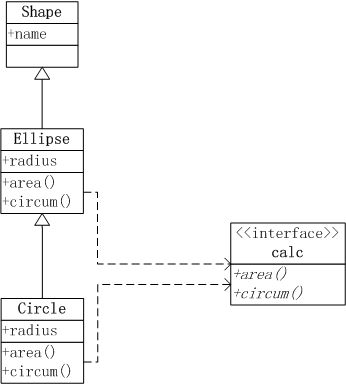``````
# 基类Shape
> setClass("Shape",slots=list(name="character",shape="character"))

# Ellipse继承Shape

# Circle继承Ellipse

# 定义area接口
> setGeneric("area",function(obj,...) standardGeneric("area"))
 "area"

# 定义area的Ellipse实现
> setMethod("area","Ellipse",function(obj,...){
+     cat("Ellipse Area :\n")
+ })
 "area"

# 定义area的Circle实现
> setMethod("area","Circle",function(obj,...){
+     cat("Circle Area :\n")
+ })
 "area"

# 定义circum接口
> setGeneric("circum",function(obj,...) standardGeneric("circum"))
 "circum"

# 定义circum的Ellipse实现
> setMethod("circum","Ellipse",function(obj,...){
+     cat("Ellipse Circum :\n")
+ })
 "circum"

# 定义circum的Circle实现
> setMethod("circum","Circle",function(obj,...){
+     cat("Circle Circum :\n")
+ })
 "circum"

# 创建实例

# 计算椭圆形的面积和周长
> area(e1)
Ellipse Area :
 31.41593
> circum(e1)
Ellipse Circum :
 23.92566

# 计算圆形的面积和周长
> area(c1)
Circle Area :
 12.56637
> circum(c1)
Circle Circum :
 12.56637
``````

6.3 任务三：增加矩形的图形处理

• 定义矩形的数据结构，并计算面积和周长
• 定义正方形的数据结构，并计算面积和周长
• 正方形是矩形的特例，定义矩形是正方形的父类，而正方形是矩形的子类。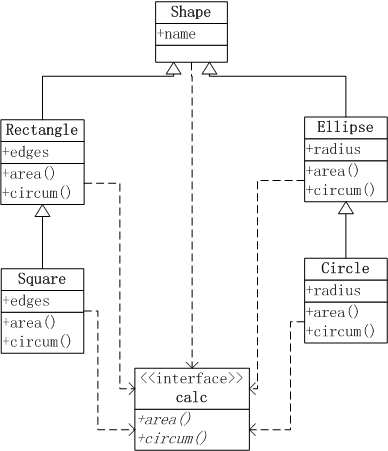``````
# 定义矩形Rectangle，继承Shape
> setClass("Rectangle",contains="Shape",slots=list(edges="numeric"),prototype=list(edges=c(1,1),shape="Rectangle"))

# 定义正方形Square，继承Rectangle
> setClass("Square",contains="Rectangle",slots=list(edges="numeric"),prototype=list(edges=1,shape="Square"))

# 定义area的Rectangle实现
> setMethod("area","Rectangle",function(obj,...){
+     cat("Rectangle Area :\n")
+     prod(obj@edges)
+ })
 "area"

# 定义area的Square实现
> setMethod("area","Square",function(obj,...){
+     cat("Square Area :\n")
+     obj@edges^2
+ })
 "area"

# 定义circum的Rectangle实现
> setMethod("circum","Rectangle",function(obj,...){
+     cat("Rectangle Circum :\n")
+     2*sum(obj@edges)
+ })
 "circum"

# 定义circum的Square实现
> setMethod("circum","Square",function(obj,...){
+     cat("Square Circum :\n")
+     4*obj@edges
+ })
 "circum"

# 创建实例
> r1<-new("Rectangle",name="r1",edges=c(2,5))
> s1<-new("Square",name="s1",edges=2)

# 计算矩形形的面积和周长
> area(r1)
Rectangle Area :
 10
> area(s1)
Square Area :
 4

# 计算正方形的面积和周长
> circum(r1)
Rectangle Circum :
 14
> circum(s1)
Square Circum :
 8
``````

6.4 任务四：在基类Shape中，增加shape属性和getShape方法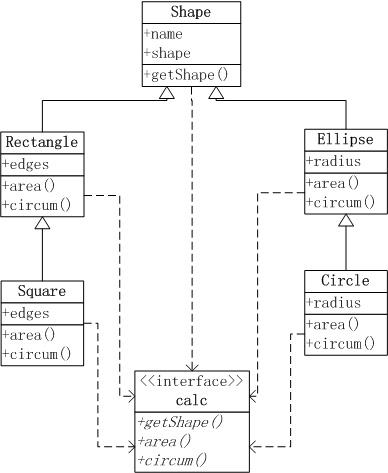``````
# 重新定义基类Shape，增加shape属性
> setClass("Shape",slots=list(name="character",shape="character"))

# 定义getShape接口
> setGeneric("getShape",function(obj,...) standardGeneric("getShape"))
 "getShape"

# 定义getShape实现
> setMethod("getShape","Shape",function(obj,...){
+     cat(obj@shape,"\n")
+ })
 "getShape"
``````

``````
# 实例化一个Square对象，并给shape属性赋值
> s1<-new("Square",name="s1",edges=2, shape="Square")

# 调用基类的getShape()函数
> getShape(r1)
Rectangle
``````

``````
setClass("Rectangle",contains="Shape",slots=list(edges="numeric"),prototype=list(edges=c(1,1),shape="Rectangle"))
setClass("Square",contains="Rectangle",slots=list(edges="numeric"),prototype=list(edges=1,shape="Square"))
``````

``````
# 实例化一个Square对象
> s1<-new("Square",name="s1",edges=2)

# 调用基类的getShape()函数
> getShape(r1)
Rectangle
``````

``````
setClass("Shape",slots=list(name="character",shape="character"))
setClass("Rectangle",contains="Shape",slots=list(edges="numeric"),prototype=list(edges=c(1,1),shape="Rectangle"))
setClass("Square",contains="Rectangle",slots=list(edges="numeric"),prototype=list(edges=1,shape="Square"))

setGeneric("getShape",function(obj,...) standardGeneric("getShape"))
setMethod("getShape","Shape",function(obj,...){
cat(obj@shape,"\n")
})

setGeneric("area",function(obj,...) standardGeneric("area"))
setMethod("area","Ellipse",function(obj,...){
cat("Ellipse Area :\n")
})
setMethod("area","Circle",function(obj,...){
cat("Circle Area :\n")
})
setMethod("area","Rectangle",function(obj,...){
cat("Rectangle Area :\n")
prod(obj@edges)
})
setMethod("area","Square",function(obj,...){
cat("Square Area :\n")
obj@edges^2
})

setGeneric("circum",function(obj,...) standardGeneric("circum"))
setMethod("circum","Ellipse",function(obj,...){
cat("Ellipse Circum :\n")
})
setMethod("circum","Circle",function(obj,...){
cat("Circle Circum :\n")
})
setMethod("circum","Rectangle",function(obj,...){
cat("Rectangle Circum :\n")
2*sum(obj@edges)
})
setMethod("circum","Square",function(obj,...){
cat("Square Circum :\n")
4*obj@edges
})

r1<-new("Rectangle",name="r1",edges=c(2,5))
s1<-new("Square",name="s1",edges=2)

area(e1)
area(c1)
circum(e1)
circum(c1)

area(r1)
area(s1)
circum(r1)
circum(s1)
``````

http://blog.fens.me/r-class-s4/## R语言面向对象编程

R的极客理想系列文章，涵盖了R的思想，使用，工具，创新等的一系列要点，以我个人的学习和体验去诠释R的强大。

R语言作为统计学一门语言，一直在小众领域闪耀着光芒。直到大数据的爆发，R语言变成了一门炙手可热的数据分析的利器。随着越来越多的工程背景的人的加入，R语言的社区在迅速扩大成长。现在已不仅仅是统计领域，教育，银行，电商，互联网….都在使用R语言。

• 张丹(Conan), 程序员Java,R,PHP,Javascript
• weibo：@Conan_Z
• blog: http://blog.fens.me
• email: bsspirit@gmail.com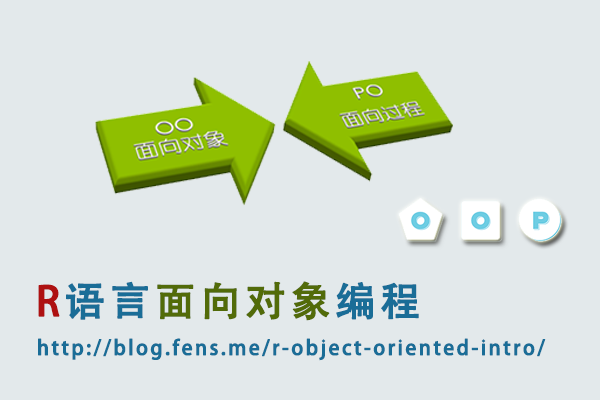1. 什么是面向对象？
2. R为什么要进行面向对象编程?
3. R的面向对象编程
4. 与其他语言的对比

## 1 什么是面向对象？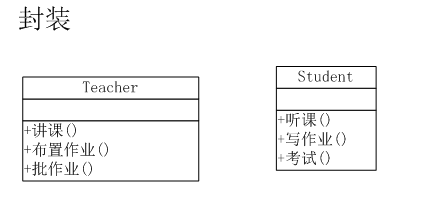• 老师的行为：讲课，布置作业，批作业
• 学生的行为：听课，写作业，考试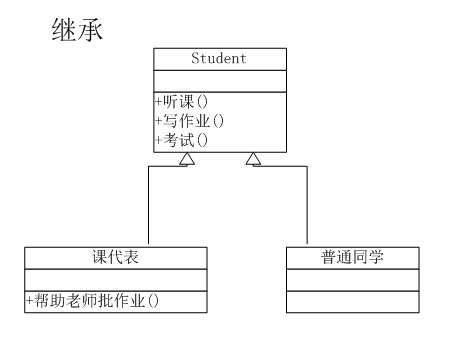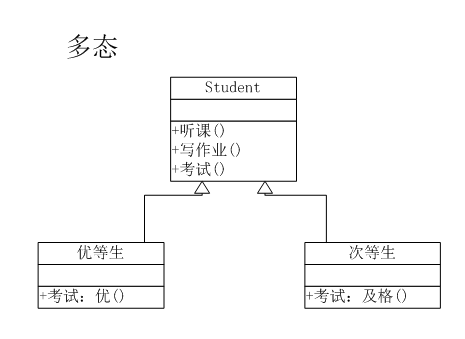is a 和 has a

• is a: 为继承关系，比如 菱形、圆形和方形都是一种形状
• has a：为组合关系或聚合关系，比如 电脑是由显示器、CPU、硬盘等组成

## 2 R为什么要进行面向对象编程?

R主要面向统计计算，而且代码量一般不会很大，几十行，几百行，使用面向过程的编程方法就可以很好地完成编程的任务。

## 3 R的面向对象编程

R的面向对象编程是基于泛型函数(generic function)的，而不是基于类层次结构。接下来，我从面向对象的3个特征入手，分别用R语言进行实现，使用的案例为上文中，老师和学生的3幅图。

3.1 R语言实现封装

``````
# 定义老师对象和行为
> teacher <- function(x, ...) UseMethod("teacher")
> teacher.lecture <- function(x) print("讲课")
> teacher.assignment <- function(x) print("布置作业")
> teacher.correcting <- function(x) print("批改作业")
> teacher.default<-function(x) print("你不是teacher")

# 定义同学对象和行为
> student <- function(x, ...) UseMethod("student")
> student.attend <- function(x) print("听课")
> student.homework <- function(x) print("写作业")
> student.exam <- function(x) print("考试")
> student.default<-function(x) print("你不是student")

# 定义两个变量，a老师和b同学
> a<-'teacher'
> b<-'student'

# 给老师变量设置行为
> attr(a,'class') <- 'lecture'
# 执行老师的行为
> teacher(a)
 "讲课"

# 给同学变量设置行为
> attr(b,'class') <- 'attend'
# 执行同学的行为
> student(b)
 "听课"

> attr(a,'class') <- 'assignment'
> teacher(a)
 "布置作业"

> attr(b,'class') <- 'homework'
> student(b)
 "写作业"

> attr(a,'class') <- 'correcting'
> teacher(a)
 "批改作业"

> attr(b,'class') <- 'exam'
> student(b)
 "考试"

# 定义一个变量，既是老师又是同学
> ab<-'student_teacher'
# 分别设置不同对象的行为
> attr(ab,'class') <- c('lecture','homework')
# 执行老师的行为
> teacher(ab)
 "讲课"
# 执行同学的行为
> student(ab)
 "写作业"
``````

3.2 R语言实现继承

``````
# 给同学对象增加新的行为
> student.correcting <- function(x) print("帮助老师批改作业")

# 辅助变量用于设置初始值
> char0 = character(0)

# 实现继承关系
> create <- function(classes=char0, parents=char0) {
+     mro <- c(classes)
+     for (name in parents) {
+         mro <- c(mro, name)
+         ancestors <- attr(get(name),'type')
+         mro <- c(mro, ancestors[ancestors != name])
+     }
+     return(mro)
+ }

# 定义构造函数，创建对象
> NewInstance <- function(value=0, classes=char0, parents=char0) {
+     obj <- value
+     attr(obj,'type') <- create(classes, parents)
+     attr(obj,'class') <- c('homework','correcting','exam')
+     return(obj)
+ }

# 创建父对象实例
> StudentObj <- NewInstance()

# 创建子对象实例
> s1 <- NewInstance('普通同学',classes='normal', parents='StudentObj')

# 给课代表，增加批改作业的行为
> attr(s2,'class') <- c(attr(s2,'class'),'correcting')

# 查看普通同学的对象实例
> s1
 "普通同学"
attr(,"type")
 "normal"     "StudentObj"
attr(,"class")
 "homework"   "attend" "exam"

# 查看课代表的对象实例
> s2
 "课代表"
attr(,"type")
attr(,"class")
 "homework"   "attend" "exam"       "correcting"
``````

3.3 R语言实现多态

``````
# 创建优等生和次等生，两个实例
> e1 <- NewInstance('优等生',classes='excellent', parents='StudentObj')
> e2 <- NewInstance('次等生',classes='poor', parents='StudentObj')

# 修改同学考试的行为，大于85分结果为优秀，小于70分结果为及格
> student.exam <- function(x,score) {
+     p<-"考试"
+     if(score>85) print(paste(p,"优秀",sep=""))
+     if(score<70) print(paste(p,"及格",sep=""))
+ }

# 执行优等生的考试行为，并输入分数为90
> attr(e1,'class') <- 'exam'
> student(e1,90)
 "考试优秀"

# 执行次等生的考试行为，并输入分数为66
> attr(e2,'class') <- 'exam'
> student(e2,66)
 "考试及格"
``````

## 4 R的面向过程编程

4.1 定义老师和同学两个对象和行为

``````
# 辅助变量用于设置初始值
> char0 = character(1)

# 定义老师对象和行为
> teacher_fun<-function(x=char0){
+     if(x=='lecture'){
+         print("讲课")
+     }else if(x=='assignment'){
+         print("布置作业")
+     }else if(x=='correcting'){
+         print("批改作业")
+     }else{
+         print("你不是teacher")
+     }
+ }

# 定义同学对象和行为
> student_fun<-function(x=char0){
+     if(x=='attend'){
+         print("听课")
+     }else if(x=='homework'){
+         print("写作业")
+     }else if(x=='exam'){
+         print("考试")
+     }else{
+         print("你不是student")
+     }
+ }

# 执行老师的一个行为
> teacher_fun('lecture')
 "讲课"

# 执行同学的一个行为
> student_fun('attend')
 "听课"
``````

4.2 区别普通同学和课代表的行为

``````
# 重定义同学的函数，增加角色判断
> student_fun<-function(x=char0,role=0){
+     if(x=='attend'){
+         print("听课")
+     }else if(x=='homework'){
+         print("写作业")
+     }else if(x=='exam'){
+         print("考试")
+     }else if(x=='correcting'){
+         if(role==1){#课代表
+             print("帮助老师批改作业")
+         }else{
+             print("你不是课代表")
+         }
+     }else{
+         print("你不是student")
+     }
+ }

# 以普通同学的角色，执行课代表的行为
> student_fun('correcting')
 "你不是课代表"

# 以课代表的角色，执行课代表的行为
> student_fun('correcting',1)
 "帮助老师批改作业"
``````

4.3 参加考试，以成绩区别出优等生和次等生

``````
# 修改同学的函数定义，增加考试成绩参数
> student_fun<-function(x=char0,role=0,score){
+     if(x=='attend'){
+         print("听课")
+     }else if(x=='homework'){
+         print("写作业")
+     }else if(x=='exam'){
+         p<-"考试"
+         if(score>85) print(paste(p,"优秀",sep=""))
+         if(score<70) print(paste(p,"及格",sep=""))
+     }else if(x=='correcting'){
+         if(role==1){#课代表
+             print("帮助老师批改作业")
+         }else{
+             print("你不是课代表")
+         }
+     }else{
+         print("你不是student")
+     }
+ }

# 执行考试函数，考试成绩为大于85分，为优等生
> student_fun('exam',score=90)
 "考试优秀"

# 执行考试函数，考试成绩为小于70分，为次等生
> student_fun('exam',score=66)
 "考试及格"
``````